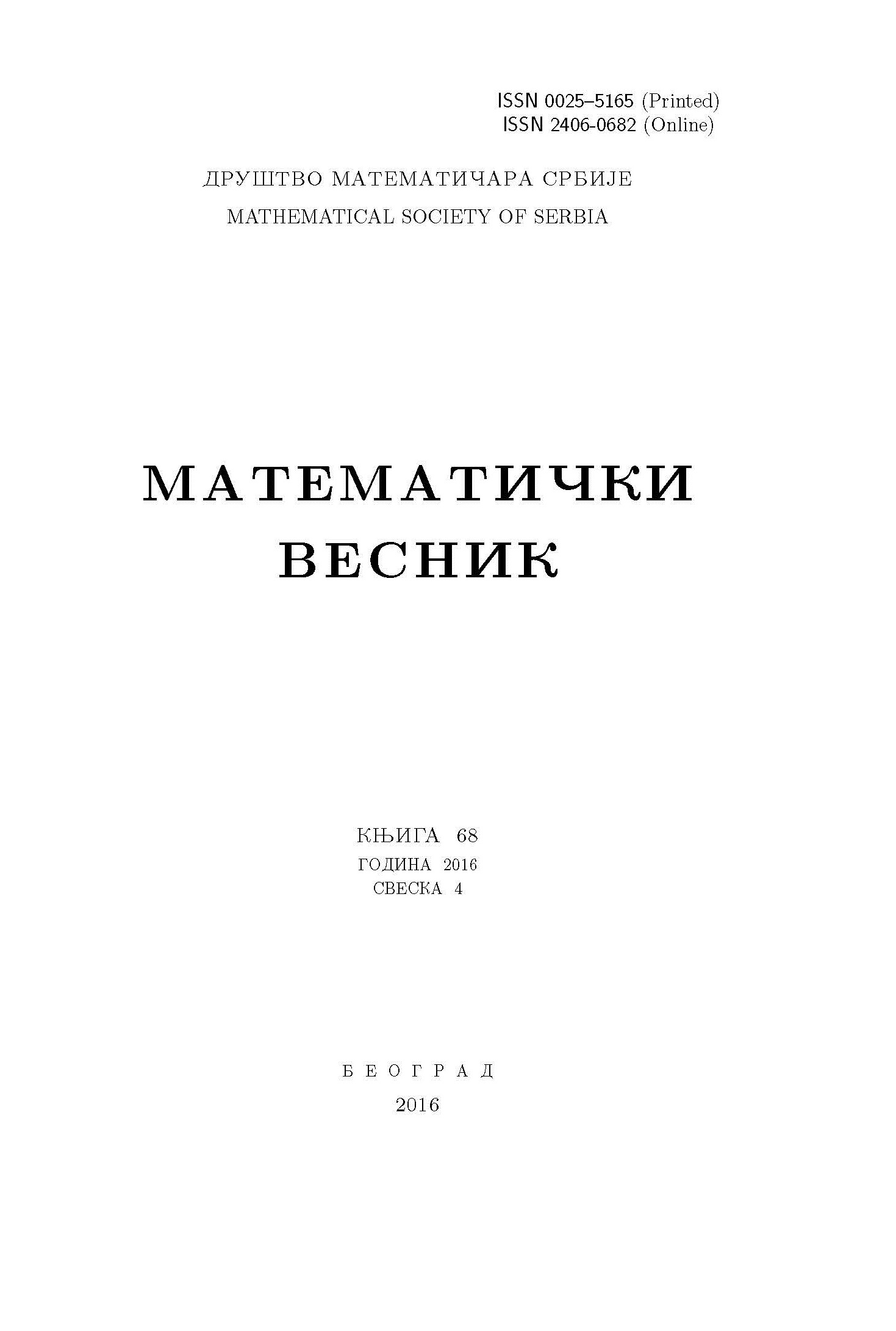﻿ Matematički Vesnik ﻿
 MATEMATIČKI VESNIK МАТЕМАТИЧКИ ВЕСНИКGENERALIZED $\boldsymbol{V}$-$\boldsymbol{Ric}$ VECTOR FIELDS ON CONTACT PSEUDO-RIEMANNIAN MANIFOLDS V. Venkatesha, H. Aruna Kumara, D. M. Naik AbstractIn this paper, we study contact pseudo-Riemannian manifold $M$ admitting generalized $V$-$Ric$ vector field. Firstly, for pseudo-Riemannian manifold, it is proved that $V$ is an infinitesimal harmonic transformation if $M$ admits $V$-$Ric$ vector field. Secondly, we prove that an $\eta$-Einstein $K$-contact pseudo-Riemannian manifold admitting a generalized $V$-$Ric$ vector field is either Einstein or has scalar curvature $r=\frac{2n\varepsilon(2n-1)}{4n-1}$. Finally, we consider a contact pseudo-Riemannian $(\kappa,\mu)$-manifold with a generalized $V$-$Ric$ vector field.Keywords: Contact pseudo-Riemannian manifolds; genaralized $V$-$Ric$ vector field; $K$-contact pseudo-Riemannian manifold; Einstein manifold. MSC: 53C50, 53C25, 53B30, 53C24 Pages:  1$-$9

﻿